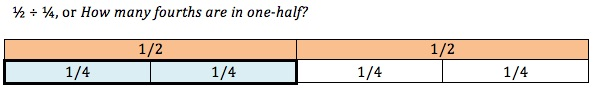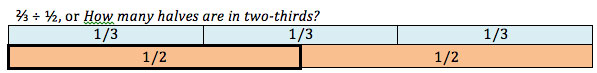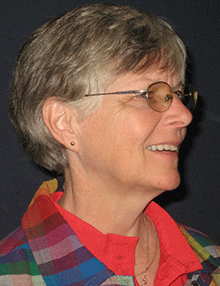## Why Do We Need Adult Education Classes?

by Donna Curry

The other day I was online, looking for an explanation about why a particular math procedure works. I knew how to do the problem, but I wanted to know why it worked. It took me quite a while to discover the answer, even though I tried some of my favorite go-to sites right away.

In the process of looking for an answer, I realized that there are MANY sites that I could go to for help if I didn’t remember the procedure, or algorithm. There are plenty of YouTube videos, each explaining the same procedure using different casts of characters. And, of course, there’s Khan Academy where you can find out how to do just about any procedure you want. So, if there are so many websites readily available to teach “math”, why do we still need adult education math classes?

That’s a question that needs answering, especially when federal and state dollars are used to fund programs. I think the answer is that those of us in adult education try to do more than just teach procedures that students have already been “taught” ad nauseam – sometimes from second grade on. What we can do that Khan doesn’t is to help students develop the conceptual understanding that underlies all those rules that students keep forgetting. I think we can also provide concrete examples from their own personal lives of math in action so that there is a link between “school math” and real-life math.

For example, think about the skill of dividing fractions by fractions. I know how to do that – it’s a piece of cake! Simply invert the second fraction and then multiply across. If I forget, I can go to myriad websites where I can be readily reminded of the procedure. But, if it’s such a simple thing, why do students still struggle?

Here’s where the role of an adult education instructor is critical. In a math classroom, a teacher can engage students in conversations about what it means to divide fractions – in fact, what it means to divide at all! (We make assumptions that students know what the four operations mean, but many of our students only know how to follow procedures related to those operations.) A teacher can begin a discussion about what it means to divide by using whole numbers (the conceptual understanding for division is the same for fractions as it is for whole numbers). She may pose the question, “What are we asking when divide 12 by 4?” (We are asking how many clumps of 4 are in 12; or we could also be asking how many will everyone get if we share 12 things among 4 people.)

If we understand that 12 ÷ 4 is asking how many 4s there are in 12, then we can ask the same thing with fractions and whole numbers. 2 ÷ ¼ is simply asking how many fourths are there in 2. Obviously, since ¼ is much smaller than 1, there are several of them in 2. There are four in 1 whole, so there are eight in two wholes. The same understanding holds true for fractions divided by fractions: ½ ÷ ¼ is still asking how many fourths are in ½. If the teacher has previously done a lot of work helping her students understand benchmark fractions, students know right off that there are two fourths in ½. Do the procedure yourself and see if that reasoning isn’t correct. And, she can actually help her students understand this idea by using visual representations.In fact, the teacher can do for students what the ubiquitous procedural explanations can’t do – she can help them learn to reason. So, to continue from the example above, if a student is faced with this problem: ⅔ ÷ ½, he can reason about the situation (thanks to the teacher’s modeling of how to do so many, many times in each class). The student can think, “Hmmm, this is asking how many ½s there are in ⅔… I know that ⅔ is larger than ½, but smaller than 1. So, I know that I could get at least one ½ out of ⅔ of something and I’d have some left over. So, I know it’s more than 1 but less than 2.”Then, and only after there has been some reasoning about the situation, the student can use the procedure to find the exact answer: ⅔ ÷ ½ = or 1 ⅓. The student will know that this is a reasonable answer because he has already figured out that the answer had to be “one and some more”.

These are the kinds of conversations that can go on in an adult education classroom that you will not find on the web. It’s these conversations, this style of teaching and learning, that make adult education teachers invaluable.

======================================================================Donna Curry is the Director of the SABES PD Center for Mathematics and Adult Numeracy, a project managed by the staff of the Adult Numeracy Center at TERC. She has trained teachers nationally, and has taught and administered ABE classes for over 30 years.